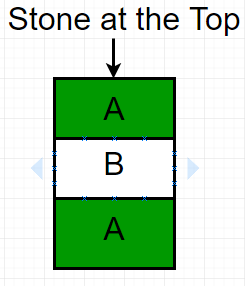# Game of Nim with removal of one stone allowed

In Game of Nim, two players take turns removing objects from heaps or the pile of stones.
Suppose two players A and B are playing the game. Each is allowed to take only one stone from the pile. The player who picks the last stone of the pile will win the game. Given N the number of stones in the pile, the task is to find the winner, if player A starts the game.

Examples :

```Input : N = 3.
Output : Player APlayer A remove stone 1 which is at the top, then Player B remove stone 2
and finally player A removes the last stone.

Input : N = 15.
Output : Player A
```

## Recommended: Please solve it on PRACTICE first, before moving on to the solution.

For N = 1, player A will remove the only stone from the pile and wins the game.
For N = 2, player A will remove the first stone and then player B remove the second or the last stone. So player B will win the game.

So, we can observe player A wins when N is odd and player B wins when N is even.

Below is the implementation of this approach:

## C++

 `// C++ program for Game of Nim with removal ` `// of one stone allowed. ` `#include ` `using` `namespace` `std; ` ` `  `// Return true if player A wins,  ` `// return false if player B wins. ` `bool` `findWinner(``int` `N) ` `{ ` `  ``// Checking the last bit of N. ` `  ``return` `N&1; ` `} ` ` `  `// Driven Program ` `int` `main() ` `{ ` `  ``int` `N = 15; ` `  ``findWinner(N)? (cout << ``"Player A"``;):  ` `                 ``(cout << ``"Player B"``;); ` `  ``return` `0; ` `}  `

## Java

 `// JAVA Code For Game of Nim with ` `// removal of one stone allowed ` `import` `java.util.*; ` ` `  `class` `GFG { ` `     `  `    ``// Return true if player A wins,  ` `    ``// return false if player B wins. ` `    ``static` `int` `findWinner(``int` `N) ` `    ``{ ` `      ``// Checking the last bit of N. ` `      ``return` `N & ``1``; ` `    ``} ` `     `  `    ``/* Driver program to test above function */` `    ``public` `static` `void` `main(String[] args)  ` `    ``{ ` `        ``int` `N = ``15``; ` `        ``if``(findWinner(N)==``1``) ` `            ``System.out.println(``"Player A"``);  ` `        ``else` `             ``System.out.println(``"Player B"``);  ` `           `  `    ``} ` `} ` ` `  `// This code is contributed by Arnav Kr. Mandal. `

## Python3

 `# Python3 code for Game of Nim with ` `# removal of one stone allowed. ` ` `  `# Return true if player A wins,  ` `# return false if player B wins. ` `def` `findWinner( N ): ` `     `  `    ``# Checking the last bit of N. ` `    ``return` `N & ``1` `     `  `# Driven Program ` `N ``=` `15` `print``(``"Player A"` `if` `findWinner(N) ``else` `"Player B"``)  ` ` `  `# This code is contributed by "Sharad_Bhardwaj". `

## C#

 `// C# Code For Game of Nim with ` `// removal of one stone allowed ` `using` `System; ` ` `  `class` `GFG { ` `     `  `    ``// Return true if player A wins,  ` `    ``// return false if player B wins. ` `    ``static` `int` `findWinner(``int` `N) ` `    ``{ ` `        ``// Checking the last bit of N. ` `        ``return` `N & 1; ` `    ``} ` `     `  `    ``/* Driver program to test above function */` `    ``public` `static` `void` `Main()  ` `    ``{ ` `        ``int` `N = 15; ` `         `  `        ``if``(findWinner(N) == 1) ` `            ``Console.Write(``"Player A"``);  ` `        ``else` `            ``Console.Write(``"Player B"``);  ` `         `  `    ``} ` `} ` ` `  `// This code is contributed by vt_m. `

## PHP

 ` `

Output :

```Player A
```

Time Complexity: O(1).

This article is contributed by Anuj Chauhan. If you like GeeksforGeeks and would like to contribute, you can also write an article using contribute.geeksforgeeks.org or mail your article to contribute@geeksforgeeks.org. See your article appearing on the GeeksforGeeks main page and help other Geeks.

My Personal Notes arrow_drop_upCheck out this Author's contributed articles.

If you like GeeksforGeeks and would like to contribute, you can also write an article using contribute.geeksforgeeks.org or mail your article to contribute@geeksforgeeks.org. See your article appearing on the GeeksforGeeks main page and help other Geeks.

Please Improve this article if you find anything incorrect by clicking on the "Improve Article" button below.

Improved By : vt_m

Article Tags :
Practice Tags :

1

Please write to us at contribute@geeksforgeeks.org to report any issue with the above content.